American Journal of Software Engineering and Applications
Volume 4, Issue 4, August 2015, Pages: 65-70

Matrix Decomposition for Recommendation System

Jie Zhu, Yiming Wei, Binbin Fu

School of Information, Beijing Wuzi University, Beijing, China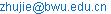(Jie Zhu)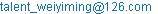(Yiming Wei)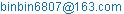(Binbin Fu)

Jie Zhu, Yiming Wei, Binbin Fu. Matrix Decomposition for Recommendation System. American Journal of Software Engineering and Applications. Vol. 4, No. 4, 2015, pp. 65-70. doi: 10.11648/j.ajsea.20150404.11

Abstract: Matrix decomposition, when the rating matrix has missing values, is recognized as an outstanding technique for recommendation system. In order to approximate user-item rating matrix, we construct loss function and append regularization constraint to prevent overfitting. Thus, the solution of matrix decomposition becomes an optimization problem. Alternating least squares (ALS) and stochastic gradient descent (SGD) are two popular approaches to solve optimize problems. Alternating least squares with weighted regularization (ALS-WR) is a good parallel algorithm, which can perform independently on user-factor matrix or item-factor matrix. Based on the idea of ALS-WR algorithm, we propose a modified SGD algorithm. With experiments on testing dataset, our algorithm outperforms ALS-WR. In addition, matrix decompositions based on our optimization method have lower RMSE values than some classic collaborate filtering algorithms.

Keywords: Matrix Decomposition, Regularization, Collaborative Filtering, Optimization

1. Introduction

In the fields of e-commerce, online video, social networks, location-based services, personalized email and advertising, recommendation system has achieved great progress and success. At least 20% of Amazon’s sales volume is benefit from recommendation system, according to its recommendation system developer .

Recommendation system can be categorized into: 1) content-based recommendation, which is based on products’ characteristics. 2) Collaborative filtering (CF), which is based on historical records of items that users have viewed, purchased, or rated.

Collaborative filtering has been widely and maturely applied in e-commerce, as CF only needs user-item matrix, which records users rating information on items . There are three primary approaches to facilitate CF algorithm: nearest neighbor model , matrix decomposition , and graph theory .

2. Rating Matrix

In this paper, Rij records the rating information of user i on item j, and rij is prediction value. Higher value means stronger preference. For example, table 1 shows a rating matrix that records five users rating information on six items. As shown in table 1, the horizontal axis shows each item, and the vertical axis shows each user.

Table 1. User-Item rating matrix.

 User_ID Item_ID Item1 Item2 Item3 Item4 Item5 Item6 User1 3 4 3 5 User2 4 2 User3 3 User4 2 4 User5 3 5 1

The degree of missing values can be measured by equation (1)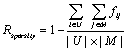(1)

|U| is the number of users, and |M| is the number of items. If the value of Rij is missing, the value of fij is 0. When the value of Rij has value, the value of fij is 1.

Collaborative filtering algorithm makes recommendation based on similarity calculation, which completely rely on the values of user-item rating matrix. Thus, recommendation accuracy is greatly affected by the sparsity of the rating matrix.

3. Matrix Factorization

In the fields of data mining and machine learning, matrix decomposition is used to approximate the original user-item rating matrix with low-level matrix. In this way, the missing value problem of recommendation system is solved. Thus, matrix factorization become a famous preprocessing step for collaborate filtering algorithm.

In the early stage of recommendation field, there are many approaches to conduct matrix factorization: probabilistic latent semantic analysis (pLSA) , neural networks, latent dirichlet allocation (LDA) , and the singular value decomposition (SVD) , and so on.

The basic idea of Singular value decomposition algorithm is using low-level matrix to replace original matrix. As there are several disadvantages of SVD in practical application, many modified algorithms are merged .

The academia proposes the latent factor model (LFM) algorithm, which is also named as implicit semantic model. Rating matrix R is decomposed into the form of matrix R =PT *Q.

This approach maps both users and items into a latent feature space. Latent factors, though not directly measurable, often contains some useful abstract information. The affinity between users and items are defined by latent factor vectors. Take figure 2 for example, class can be explained as latent factor. P is user factor matrix, and Q is item factor matrix.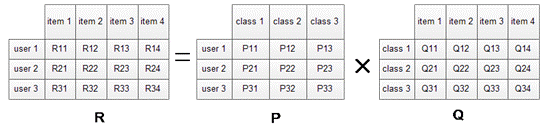Figure 1. Latent factor model (LFM).

3.1. Loss Function

In order to accurately approximate the original user-item rating matrix, we construct loss function (2) to help finding proper user matrix and item matrix. The initialization of user matrix and item matrix are often using random number, and are proportional with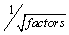by experience.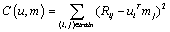(2)

To prevent overfitting, we add regularization constraint to (2). Thus, the loss function becomes an optimization problem. Many past studies have proposed optimization methods to solve (3), e.g.  . Among them, alternating least squares (ALS) and stochastic gradient descent (SGD) are popularly used.(3)

In (3), f is the number of latent factor, u is user factor matrix, and m is item factor matrix.

3.2. Alternating Least Squares with Weighted Regularization

The method of alternative least squares is originated from mathematical optimization technique. In the loss function, u and m are unknown. The basic idea of ALS-WR is assume that one unknown parameter is certain. Then the loss function can be regard as a least squares problem. After setting a stopping criterion, we can get a proper m or u with iteration method.

In addition, we use Tikhonov regularization to punish excessive parameters . Thus, (3) is updated into (4), which is based on the weighted regularization of alternative least squares method (ALS-WR):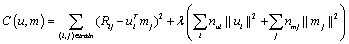(4)

Input: original rating matrix Rij, the number of feature f

Output: prediction matrix rij

Step 1: initial matrix m by assigning the average ratings as the value of first row, and complete remaining part of the matrix with small random numbers.

Step 2: fix item factor matrix, and calculate the derivative of function (4)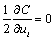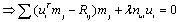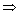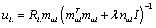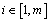Step3: fix user factor matrix, and calculate the derivative of function (4)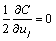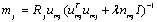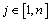Step4: Repeat step2 and 3, until a stopping criterion is satisfied (enough iterations or the result of equation (2) is converged), and return u and m.

Step5: Calculate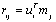When recommendation system need parallel computations, ALS-WR has high accuracy and stronger scalability. As in this algorithm, system can calculate item factor matrix independently without consideration of user factor matrix, or calculate user factor matrix independently without consideration of item factor matrix. Thus, there is high efficient in solving loss function (4).

Stochastic gradient descent (SGD) is based on gradient descent. The update rule of gradient descent is taking steps proportional to the negative of the gradient (or of the approximate gradient) of loss function at the current point. The basic idea of SGD is that, instead of expensively calculating the gradient of instance point in loss function (3), it randomly selects a Rij entry from (3) and calculates the corresponding gradient. When the number iteration increase, user factor ui and item factor mj are updated by the following rules(5)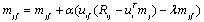(6)

In the rules of (5) and (6), Parameter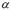is learning rate. Its value is generally obtained by trial and error approach until the training model converges. Parameter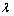is regularization coefficient for avoiding over-fitting.

Within each iteration process, user factor ui and item factor mj are updated by calculating gradient descent value of a sequence of rating instances. R(u) is a rating set of users, and each training instance is recorded in ( ru1, ru2, ru3, … ruk) .We use u(0) to represent the initial value of user factor uif , and u(1) represent updated value after calculating instance ru1.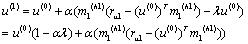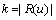(7)

In (7), h1 represent the temporary state of m1. After training instance from ru2 to ruk, (7) will be updated into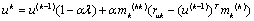(8)

After one round of iteration,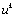can be rewrite into (9). We use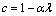in (9).(9)

In (9),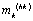represent the temporary state of mk using instance ruk. In the same way, we use R(m) to represent rating set of items. The set of (r1m, r2m, r3m, … rhm) records the sequences of instances that are select to calculate gradient descent (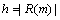).After one round of iteration,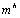can be rewrite into(10)

Considering (9) and (10), we can see user factor ui and item factor mj are not only depend on initial value, but also depend on temporary states ofand. From the above iteration processes and update rules from (5) to (10), we can see that SGD is a serialization method.

4. Our Approach

As the gradient search processes traverse the entire rating instances, it can update user and item factor matrixes in every round of iteration. Thus, SGD can accurately describe the multiple features of the rating matrix. Matrix decompositions based on SGD have high precision of recommendation, and strong scalability.

However, the iteration process of SGD is depending on temporary state of item factor matrix m and user factor matrix u, and the update rules need the accumulated value of each iteration process in a serial mode. Thus, in some cases the training processes may experiences system deadlocks. In addition, in the multi-core environment, SGD method is low efficiency. In the contrary, ALS-WR algorithm doesn’t have such problems, as it can update m and u independently.

Based on the advantage of ALS-WR, we modify SGD, and propose a parallel SGD algorithm (PSGD). The following is the training process of matrix factorization based on PSGD:

Input: original rating matrix Rij, the number of feature f Initial: matrix m and u

While stopping criterion is not satisfied

For each user

Do Allocate a new thread for user

For each train rating instance

Do Calculate loss function

Update matrix u

End for

End for

For each item

Do Allocate a new thread for user

For each train rating instances

Do Calculate loss function

Update matrix m

End for

End for

Output: updated latent factor matrix u and m

In above algorithm, the update rules are (5) and (6). Instance training processes are based on (8) or (9). As we can see from PSGD algorithm, the matrix u and m can be update separately. Thus, the rate of convergence will be accelerated, and the results will be more accurate.

5. Experimental Results of ALS-WR and PSGD

The accuracy of the recommendation model is measured by root mean square error (RMSE), and |train| represents the validation dataset. A lower value of RMSE indicates a higher accurate in recommendation system.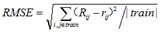Apache Mahout is a project of the Apache Software Foundation to produce free implementations of distributed or otherwise scalable machine learning algorithms focused primarily in the areas of collaborative filtering.

In this paper we focus on the collaborative filtering algorithm based matrix decomposition. Thus, in order to simulate the real recommendation system environment, we run our experiment in Mahout Environment, which has been maturely adopted in a distribute Hadoop system in many commercial fields.

Our experiment uses the famous MovieLens datasets which is developed by the GroupLens Lab of Minnesota University. Our matrix factorization algorithms are test on MovieLens 100k dataset, which including 100,000 records of rating information that are given by 943 users on 1682 movies. The sparsity of dataset is 6.305%.

In the following experiments, MovieLens dataset is divided into two parts. 70% of it is training set, and the rest is testing set. In the following comparison, there are three parameters: number of hidden features, overfitting parameter and number of iterations. The parameter selections are derived by experience and cross validation. In the following experiments, the default settings are: 0.05(overfitting parameter), 20(number of iterations) and 30(number of hidden features)

5.1. Experiment 1

In figure 2, the horizontal axis represents the number of hidden features in LFM algorithm, and the vertical axis records values of RMSE. When the number of hidden feature is changing from 15 to 75, the RMSE value of ALS-WR is obviously higher than PSGD. In addition, with the increase of hidden features, the prediction matrix tends to be dense and have less missing values in user-item matrix. Thus, the RMSE values of them reach a stabilized state.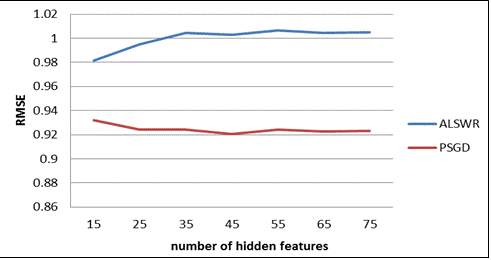Figure 2. Comparison of ALS-WR and PSGD on hidden features.

In figure 3, the horizontal axis records the changes of overfitting parameter in loss function (3), and the vertical axis shows RMSE values. When the value of overfitting parameter is on the increase, the RMSE values of ALS-WR and PSGD are closer. From the overall look, the RMSE curve of ALS-WR is higher than PSGD.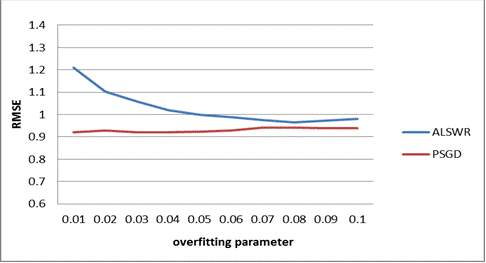Figure 3. Comparison of ALS-WR and PSGD on overfitting parameter.

In figure 4, the horizontal axis shows the iteration numbers of matrix decomposition algorithm based on ALS-WR and PSGD optimization methods, and the vertical axis shows RMSE values. When the number of iterations is increasing from 10 to 80, the RMSE value of ALS-WR is high than PSGD. In addition, with the increase of iteration number, both optimization algorithms tend to convergence, and their RMSE are gradually stabilized.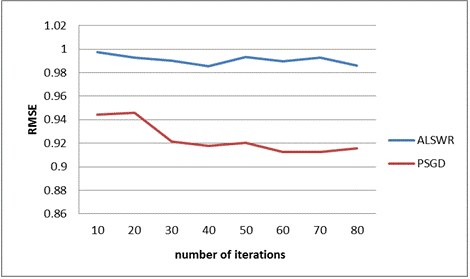Figure 4. Comparison of ALS-WR and PSGD on number of iterations.

5.2. Experiment 2

In this experiment, we use the same datasets as experiment 1. Userbased and itembased are classic collaborate filtering algorithms, which are based on user or item similarity. SlopeOne algorithm uses linear regression to filter message and make recommendation. BiasMF is a modified version of LFM, with considering the influence of system inherent factors. For LFM and BiasMF, they are matrix decomposition algorithms. In the following experiment, LFM and BiasMF will be solved using PSGD method.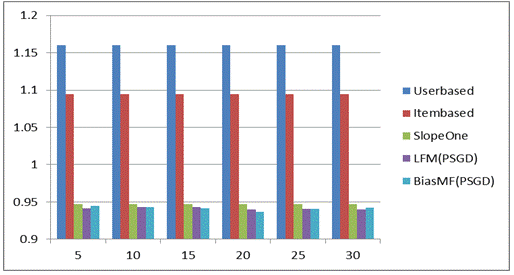Figure 5. Comparison of algorithms on hidden feature.

In figure 5, Userbased, Itembased and SlopeOne algorithms are not matrix decomposition methods. Thus, their RMSE values are remaining certain.

According to the chart, the RMSE value of LFM(PSGD) and BiasMF(PSGD) are obviously lower than classic collaborate filtering algorithms of Userbased and itembased, and slightly lower than SlopeOne algorithms.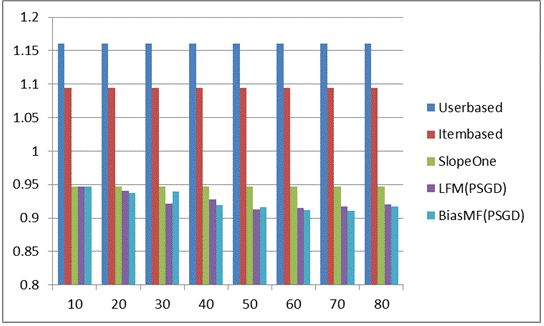Figure 6. Comparison of algorithms on number of iterations.

In figure 6, with the number of iteration increases, their RMSE values tend to be stabilized. As we can see from figure 6, matrix decomposition based PSGD algorithms have better performances than classic collaborate filtering algorithms.

6. Conclusion

In this paper, we introduced recommendation system and collaboration filtering algorithm. When user-item rating matrix is sparse, matrix factorization is recognized as an efficient approach to approximate original rating matrix. Latent factor model (LFM) is a famous decomposition method, which related user and item to their implicit features. In order to get an accurate and proper prediction matrix, we construct loss function.

The loss function is an optimize problem, and alternating least squares with weighted regularization (ALS-WR) is an efficient and parallel method to solve it. However, the calculation of matrix decomposition based on ALS-WR involved with matrix inversion, thus it has great computation complexity.

Similar to ALS, Stochastic gradient descent (SGD) is another famous optimization approach. Calculation based on SGD is needs to obtain the gradient of each rating instance. Thus, it is easy to conduct matrix composition based on SGD. However, SGD is a sequential algorithm.

Based on the strengths of ALS-WR, we modify and propose a new algorithm PSGD based on SGD. The training processes of PSGD are twice than SGD approach, it seems to be a reduction in efficiency. However, PSGD can update user or item factor matrix independently, and thus provide the possibility of parallel performance. Based on experiment 1, PSGD has better performance than ALS-WR.

In addition, we compare two matrix decomposition algorithms based on PSGD with classic collaborate filtering algorithms. In the real recommendation system, the sparse rating matrix increases the difficulty to calculate similarity between users or items, but matrix decomposition methods are survived of this problem. As experiment 2 shows, matrix decomposition algorithms based on PSGD are out performance than collaborate filtering algorithms based on similarity.

Acknowledgements

This paper is supported by the Funding Project for Technology Key Project of Municipal Education Commission of Beijing (ID:TSJHG201310037036); Funding Project for Beijing key laboratory of intelligent logistics system ;Funding Project of Construction of Innovative Teams and Teacher Career Development for Universities and Colleges Under Beijing Municipality (ID:IDHT20130517), and Beijing Municipal Science and Technology Project (ID:Z131100005413004); Funding Project for Beijing philosophy and social science research base specially commissioned project planning (ID:13JDJGD013).

References

1. LINDEN G, SMITH B, YORK J. Amazon.com recommendations: Item-to-item collaborative filtering [J]. IEEE Internet Computing, 2003, 7(1): 76－80．
2. KOREN Y. Factorization meets the neighborhood: a multifaceted collaborative filtering model[C]//Proceedings of the 14th ACM SIGK-DD International Conference on Knowledge Discovery and Data Mining．New York: ACM, 2008: 426－434．
3. ALI K, WIJNAND V S. TiVo: Making show recommendations using a distributed collaborative filtering architecture[C]//KDD'04: Proceedings of the 10th ACM SIGKDD International Conference on Knowledge Discovery and Data Mining. New York: ACM, 2004:394- 401．
4. GOLDBERG K Y，ROEDER T，GUPTA D，et al. Eigentaste: A constant time collaborative filtering algorithm [J]. Information Retrieval, 2001, 4( 2): 133－151．
5. SALAKHUTDINOV R，MNIH A，HINTON G．Restricted Boltzmann machines for collaborative filtering[C]//Proceedings of the 24th International Conference on Machine Learning．New York: ACM, 2007:791－798．
6. HOFMANN T. Latent semantic models for collaborative filtering [J]. ACM Transactions on Information Systems，2004, 22(1) : 89－115．
7. BLEI D，NG A，JORDAN M．Latent Dirichlet allocation [J]. Journal of Machine Learning Research, 2003, 3: 993－1022．
8. DaWei C, Zhao Y, HaoYan L. The overfitting phenomenon of SVD series algorithms in rating matrix [J]. Journal of Shandong university (engineering science), 2014，44(3): 15-21
9. XiaoFeng H, Xin L, Qingsheng Z. A parallel improvements based on regularized matrix factorization of collaborative filtering model [J]. Journal of electronics and information, 2013，35(6):1507-1511.
10. Zhou Y, Wilkinson D, Schreiber R, et al. Large-scale parallel collaborative filtering for the Netflix prize [M]//Algorithmic Aspects in Information and Management. Springer Berlin Heidelberg, 2008: 337-348.
11. I.Pil´aszy,D. Zibriczky, and D.Tikk. Fast ALS-based matrix factorization for explicit and implicit feedback datasets. In Proceedings of the Fourth ACM Conference on Recommender Systems, pages71–78, 2010.

 Contents 1. 2. 3. 3.1. 3.2. 3.3. 4. 5. 5.1. 5.2. 6.
Article ToolsAbstractPDF(494K)